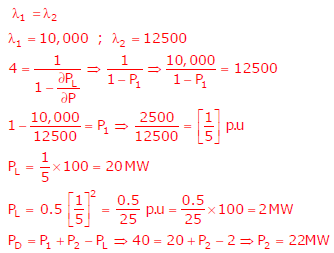# Power Systems - Online Test

Q1. A nuclear power station of 500 MW capacity is located at 300 km away from a load center. Select the most suitable power evacuation transmission configuration among the following options
Explaination / Solution:
No Explaination.

Q2. A negative sequence relay is commonly used to protect
Explaination / Solution:
No Explaination.

Q3. For enhancing the power transmission in along EHV transmission line, the most preferred method is to connect a
Explaination / Solution:Q4.
A load center of 120MW derives power from two power stations connected by 220kV transmission lines of 25km and 75km as shown in the figure below. The three generators G1,G2 and G3 are of 100MW capacity each and have identical fuel cost characteristics. The minimum loss generation schedule for supplying the 120 MW load is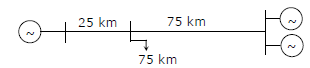Explaination / Solution:

Loss α p; Loss α length
For checking all options only option A gives less losses.

Q5.
Two generator units G1 and G2 are connected by 15 kV line with a bus at the mid-point as shown below.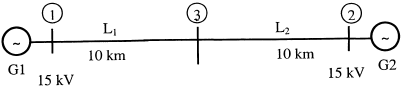G1 = 250 MVA, 15kV, positive sequence reactance X = 25% on its own base
G2 = 100 MVA, 15kV, positive sequence reactance X = 10 % on its own base
L1 and L2 = 10km, positive sequence reactance X = 0.225 Ω / km
For the above system, the positive sequence diagram with the p.u values on the MVA common base is
Explaination / Solution: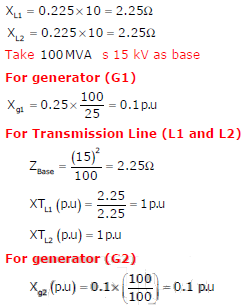Q6.
Two generator units G1 and G2 are connected by 15 kV line with a bus at the mid-point as shown below.G1 = 250 MVA, 15kV, positive sequence reactance X = 25% on its own base
G2 = 100 MVA, 15kV, positive sequence reactance X = 10 % on its own base
L1 and L2 = 10km, positive sequence reactance X = 0.225 Ω / km
In the above system, the three-phase fault MVA at the bus 3 is
Explaination / Solution: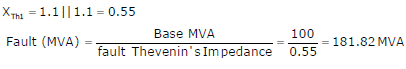Q7.
A solar energy installation utilize a three – phase bridge converter to feed energy into power system through a transformer of 400V/400 V, as shown below.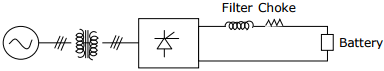The energy is collected in a bank of 400 V battery and is connected to converter through a large filter choke of resistance 10Ω.
The maximum current through the battery will be
Explaination / Solution: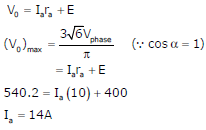Q8.
A solar energy installation utilize a three – phase bridge converter to feed energy into power system through a transformer of 400V/400 V, as shown below.The energy is collected in a bank of 400 V battery and is connected to converter through a large filter choke of resistance 10Ω.
The kVA rating of the input transformer is
Explaination / Solution: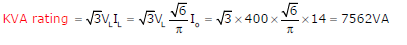Q9. The bus admittance matrix of a three-bus three-line system is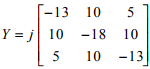If each transmission line between the two buses is represented by an equivalent π-network, the magnitude of the shunt susceptance of the line connecting bus 1 and 2 is
Explaination / Solution: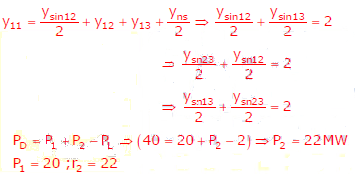Q10. The figure shows a two-generator system supplying a load of PD = 40 MW, connected at bus 2.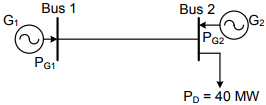The fuel cost of generators G1 and G2 are :and the loss in the line iswhere the loss coefficient is specified in pu on a 100 MVA base. The most economic power generation schedule in MW is
Explaination / Solution: